# Python数据挖掘——概要

## 一、数据挖掘过程

1.数据选择

2.数据预处理

python字符串处理（相当方便）、正则式匹配、pandas、beautifulsoup处理Html标签等等工具。

3.特征工程/数据转换

4.数据挖掘

5.解释与评价

## 二、数据挖掘常用算法简介

### 2.分类算法

CBA(Classification Based on Association)算法：基于关联规则的分类算法；

MIND(Mining in Database)算法 ：采用数据库中用户定义的函数(user-definedfunction，简称UDF)来实现分类的算法；

### 3.聚类算法

Ipartitioning method(划分方法) 给定1个N个对象或者元组的数据库，1个划分方法构建数据的K个划分，每1个划分表示1个聚簇，并且K<N。经典算法是K-MEAN(K平均值)；

hierarchical method(层次方法)

grid based method(基于网格的方法) 这种方法采用一个多分辨率的网格数据结构。将空间量化为有限数目的单元，这些单元形成了网格结构，所有聚类分析都在网格上进行。常用的算法有STING，SkWAVECLUSTER和 CLIQUE；

## 三、数据挖掘算法实现

### 1、相关知识

#### (1)距离度量：在数据挖掘中需要明确样本数据相似度，通常可以计算样本间的距离，如下为常用距离度量的介绍。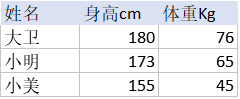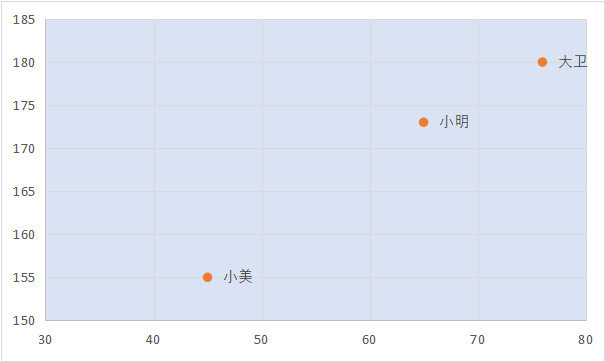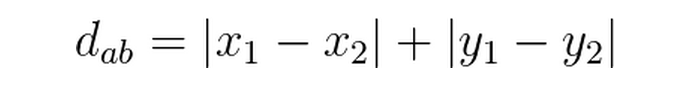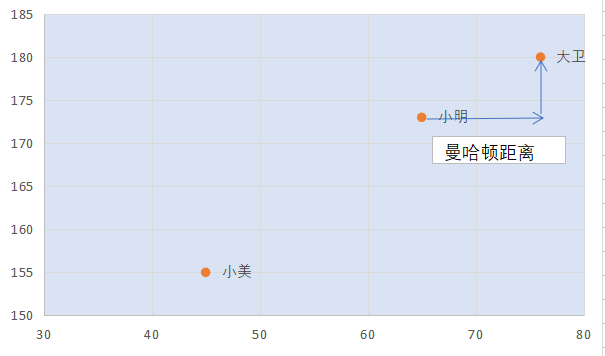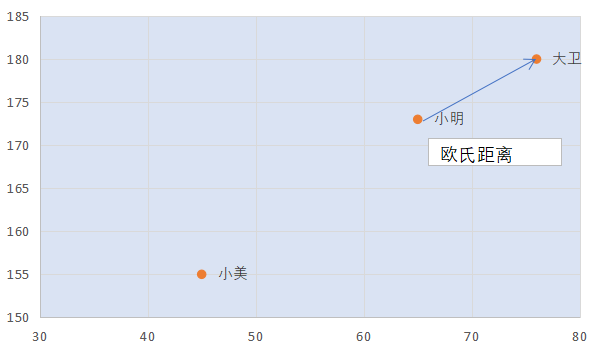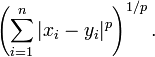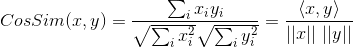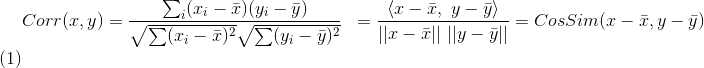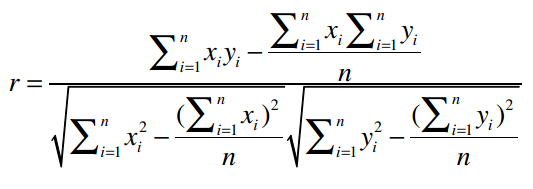#### (2)数据标准化：参考文章

min-max 标准化：将数值范围缩放到（0,1）,但没有改变数据分布。max为样本最大值，min为样本最小值。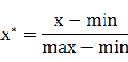z-score 标准化：将数值范围缩放到0附近, 经过处理的数据符合标准正态分布。u是平均值，σ是标准差。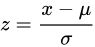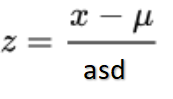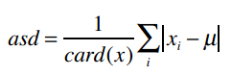#### (3) 算法的效果评估：

N折交叉验证又称为留一法：用几乎所有的数据进行训练，然后留一个数据进行测试，并迭代每一数据测试。留一法的优点是：确定性。

### 2、协同过滤推荐算法

#### 代码实现、数据集及参考论文 电影推荐——基于用户、物品的协同过滤算法

...

r = Recommendor()

print("items base协同推荐 slope one")
#items base协同推荐算法 Slope one
r.slope_one_recommendation('lyy')

print("items base协同推荐 cos")
#items base协同推荐算法  修正余弦相似度
r.cos_recommendation('lyy')

print("users base协同推荐")
#userbase协同推荐算法
r.user_base_recommendation("lyy")


#### (1)基于用户的协同推荐算法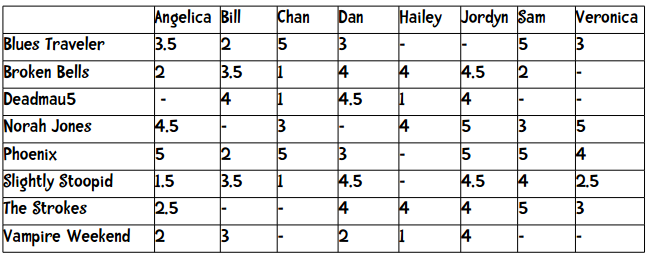#### 修正余弦相似度算法：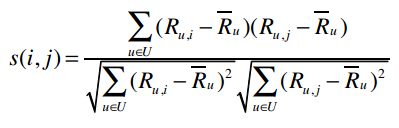#### Slope One推荐算法：

dev(i,j)为遍历所有共有物品i，j的共有用户u的评分平均差异。

card(Sj,i(X))则表示同时评价过物品j和i的用户数。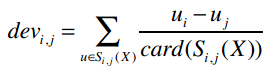PWS1(u)j表示我们将预测用户u对物品j的评分。

dev(i,j)为遍历所有共有物品i，j的共有用户u的评分平均差异。

C(ji)也就是card(Sj,i(X))表示同时评价过物品j和i的用户数。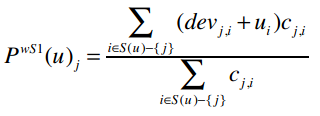Slope One算法优点：算法简单；扩展性好，只需要更新共有属性的用户评价，而不需要重新载入整个数据集。

Slope One算法的缺点：稀疏性，需要基于用户的评分数据；

### 3、分类算法

#### 代码实现 鸢尾花KNN分类算法

...

# KNN算法
def knn(self, oj_list):
weight_dict = {"Iris-setosa":0.0, "Iris-versicolor":0.0, "Iris-virginica":0.0}
for atuple in oj_list:
weight_dict[atuple] += (1.0 / atuple)
rel_class = [(key, value) for key, value in weight_dict.items()]
#print(sorted(rel_class, key=lambda x:x, reverse=True))
rel_class = sorted(rel_class, key=lambda x:x, reverse=True)
return rel_class

...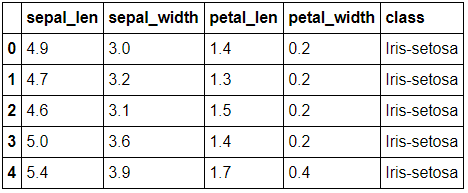KNN分类算法的缺点：无法对分类结果的置信度进行量化；是被动学习的算法，每次测试需要需要遍历所有的训练集后才能分类。

#### 代码实现 区分新闻类别朴素贝叶斯分类算法

...
def train_data(self):
#训练组的条件概率
for word in self.vocabulary:
for category,value in self.prob.items():
if word not in self.prob[category]:
count = 0
else :
count = self.prob[category][word]
#优化条件概率公式
self.prob[category][word] = (count + 1) / (self.total[category] + len(self.vocabulary))

...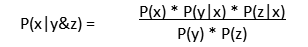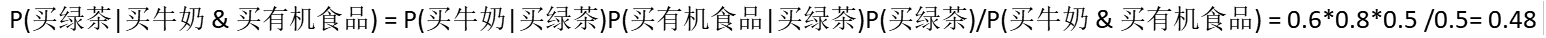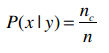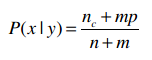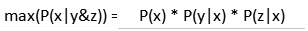#### 代码实现 区分猫的图片

...
# cost函数，计算梯度
def propagate(w, b, X, Y):
m = X.shape
A = sigmoid(np.dot(w.T, X) + b)
cost = -1 / m * np.sum(Y * np.log(A) + (1 - Y) * np.log(1 - A))
dw = 1 / m * np.dot(X, (A - Y).T)
db = 1 / m * np.sum(A - Y)
...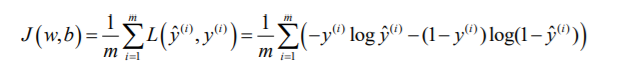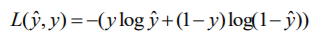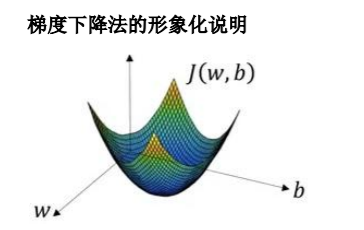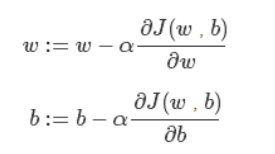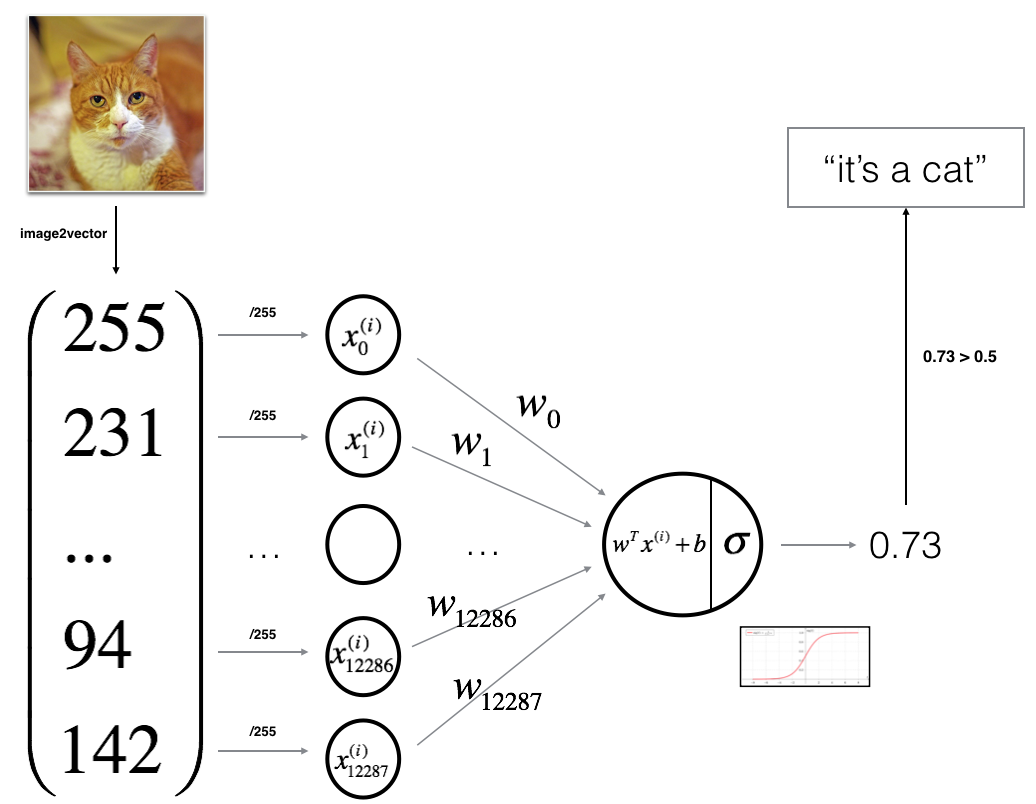### 4、聚类算法

#### 代码实现 Kmean++聚类

        ...
#kmean初始化随机k个中心点
#random.seed(1)
#center = [[self.data[i][r] for i in range(1, len((self.data)))]
#for r in random.sample(range(len(self.data)), k)]

# Kmean ++ 初始化基于距离份量随机选k个中心点
# 1.随机选择一个点
center = []
center.append(random.choice(range(len(self.data))))
# 2.根据距离的概率选择其他中心点
for i in range(self.k - 1):
weights = [self.distance_closest(self.data[x], center)
for x in range(len(self.data)) if x not in center]
dp = [x for x in range(len(self.data)) if x not in center]
total = sum(weights)
#基于距离设定权重
weights = [weight/total for weight in weights]
num = random.random()
x = -1
i = 0
while i < num :
x += 1
i += weights[x]
center.append(dp[x])
...
`

k-means++算法可概括为：

（1）基于各点到中心点得距离分量，依次随机选取到k个元素作为中心点：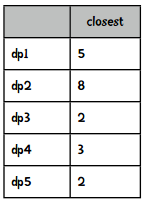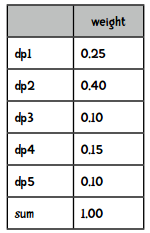（2）根据各点到中心点的距离分类；

（3）计算各个分类新的中心点。

©️2019 CSDN 皮肤主题: 技术工厂 设计师: CSDN官方博客